Question

# Two forces are applied to a tree stump to pull it out of the ground. Force...

Two forces are applied to a tree stump to pull it out of the ground. Force F with arrowA has a magnitude of 2239 newtons and points 33.0° south of east, while force F with arrowB has a magnitude of 3159 newtons and points due south. Using the component method, find the magnitude and direction of the resultant force F with arrowA + F with arrowB that is applied to the stump. Specify the direction with respect to due east.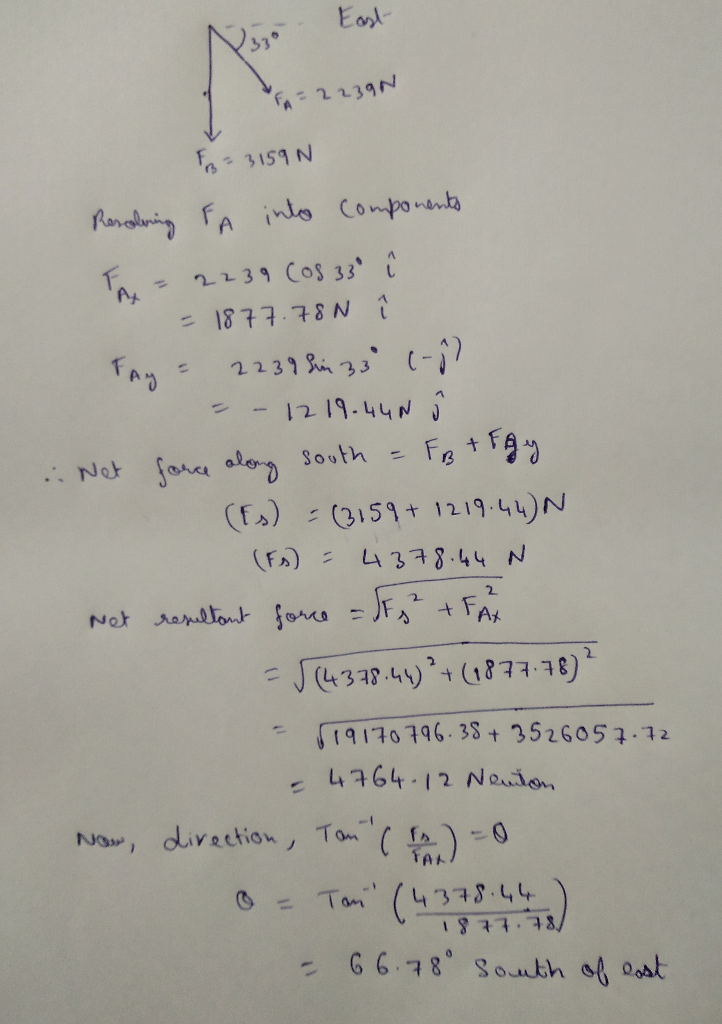#### Earn Coins

Coins can be redeemed for fabulous gifts.

Similar Homework Help Questions
• ### Two forces are applied to a tree stump to pull it out of the ground. Force...

Two forces are applied to a tree stump to pull it out of the ground. Force F with arrowA has a magnitude of 2219 newtons and points 31.0° south of east, while force F with arrowB has a magnitude of 3157 newtons and points due south. Using the component method, find the magnitude and direction of the resultant force F with arrowA + F with arrowB that is applied to the stump. Specify the direction with respect to due east.

• ### Two forces are applied to a tree stump to pull it out of the ground. Force...

Two forces are applied to a tree stump to pull it out of the ground. Force FA has a magnitude of 2200 newtons and points 34.5° south of east, while force FB has a magnitude of 3160 newtons and points due south. Using the component method, find the magnitude and direction of the resultant force FA + FB that is applied to the stump. Specify the direction with respect to due east.

• ### Two forces are applied to a tree stump to pull it out of the ground. Force FA has a magnitude of...

Two forces are applied to a tree stump to pull it out of the ground. Force FA has a magnitude of 2020 newtons and points 34.0° south of east, while force FB has amagnitude of 3160 newtons and points due south. Using the component method, find the magnitude and direction of the resultant force FA + FB that is applied to thestump. Specify the direction with respect to due east.

• ### Ttwo forces are applied to a tree stump to pull It out of the ground. Force...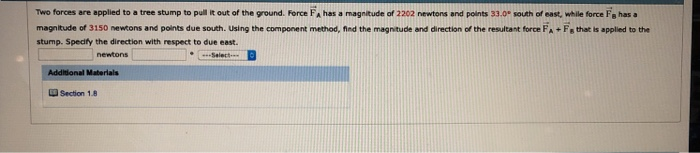Ttwo forces are applied to a tree stump to pull It out of the ground. Force FA has a magnitude of 2202 newtons and points 33.0" south of east, while force Fs has a magnitude of 3150 newtons and ports due south. Using the component method, ned the magnitude and diretos of the resuea t force A·E, t ae,ppled i' th stump. Spedfy the direction with respect to due east -Select Additional Materials Section 1.8

• ### As an aid in working this problem, consult Concept Simulation 1.1. Two forces are applied to...

As an aid in working this problem, consult Concept Simulation 1.1. Two forces are applied to a tree stump to pull it out of the ground. Force A has a magnitude of 2960 newtons (N) and points 44.0 ° south of east, while force B has a magnitude of 3820 N and points due south. Using the component method, find the (a) magnitude and (b) direction of the resultant force A + B that is applied to the stump. Specify...

• ### physics help 4

Two forces are applied to a tree stump to pull it out of the ground. Force FA has a magnitude of 2050 newtons and points 36.5° south of east, while force FB has amagnitude of 3160 newtons and points due south. Using the component method, find the magnitude and direction of the resultant force FA + FB that is applied to thestump. Specify the direction with respect to due east.

• ### Chapter 01, Problem 47 Multiple Concept Example 9 reviews the concepts that play a role in...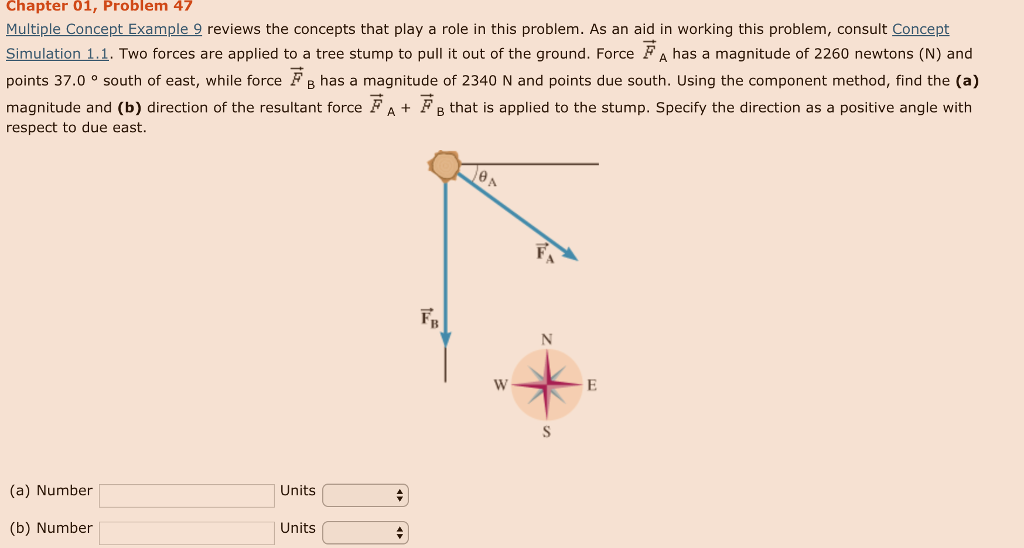Chapter 01, Problem 47 Multiple Concept Example 9 reviews the concepts that play a role in this problem. As an aid in working this problem, consult Concept Simulation 1.1. Two forces are applied to a tree stump to pull it out of the ground. Force FA has a magnitude of 2260 newtons (N) and points 37.0 ° south of east, while force FB has a magnitude of 2340 N and points due south. Using the component method, find the (a)...

• ### An ocean liner leaves New York City and travels 54.0 north of east for 163 krm....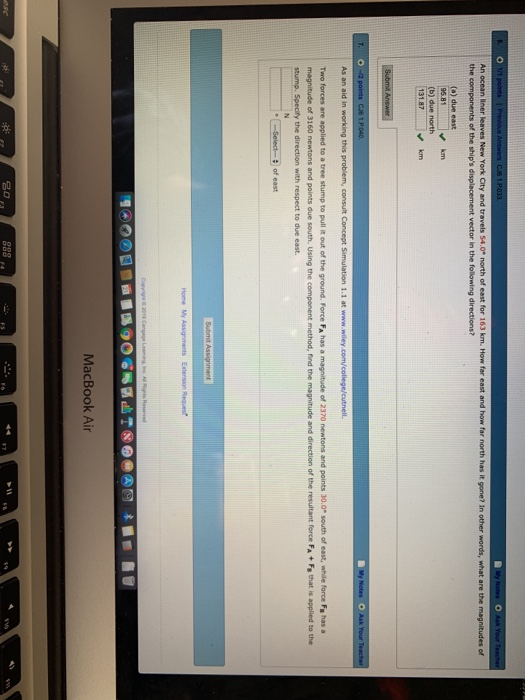An ocean liner leaves New York City and travels 54.0 north of east for 163 krm. How far east and how far north has it gone? In other words, what are the magnitudes of the components of the ship's displacement vector in the following directions? kom 95.81 (b) due north 131.87km As an aid in working this Two forces are applied to a tree stump to pull it out of the ground. Force FA has a magnitude of 2370 newtons...

• ### Cutnell, Physics, 10e College Physics (PHYC 2053 & 205 nt 9 revilews the concepts that play...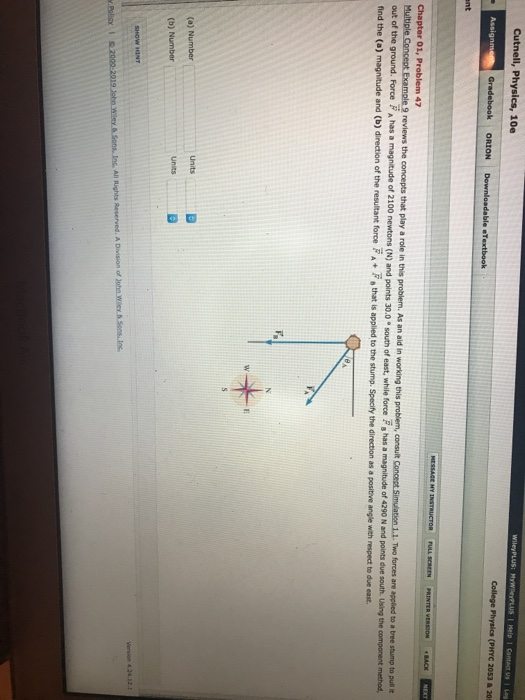Cutnell, Physics, 10e College Physics (PHYC 2053 & 205 nt 9 revilews the concepts that play a role in this problem. As an aid in working this problem, consult 1. Two forces are applied to a tree stump to pull it F, has a magnitude of 4290 N and points due south Using the component method, 30.0 south of east, while forece find the (a) magnitude and (b) direction of the resultant force A F that is applied to the...